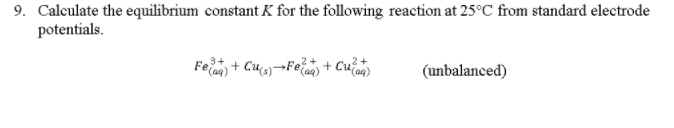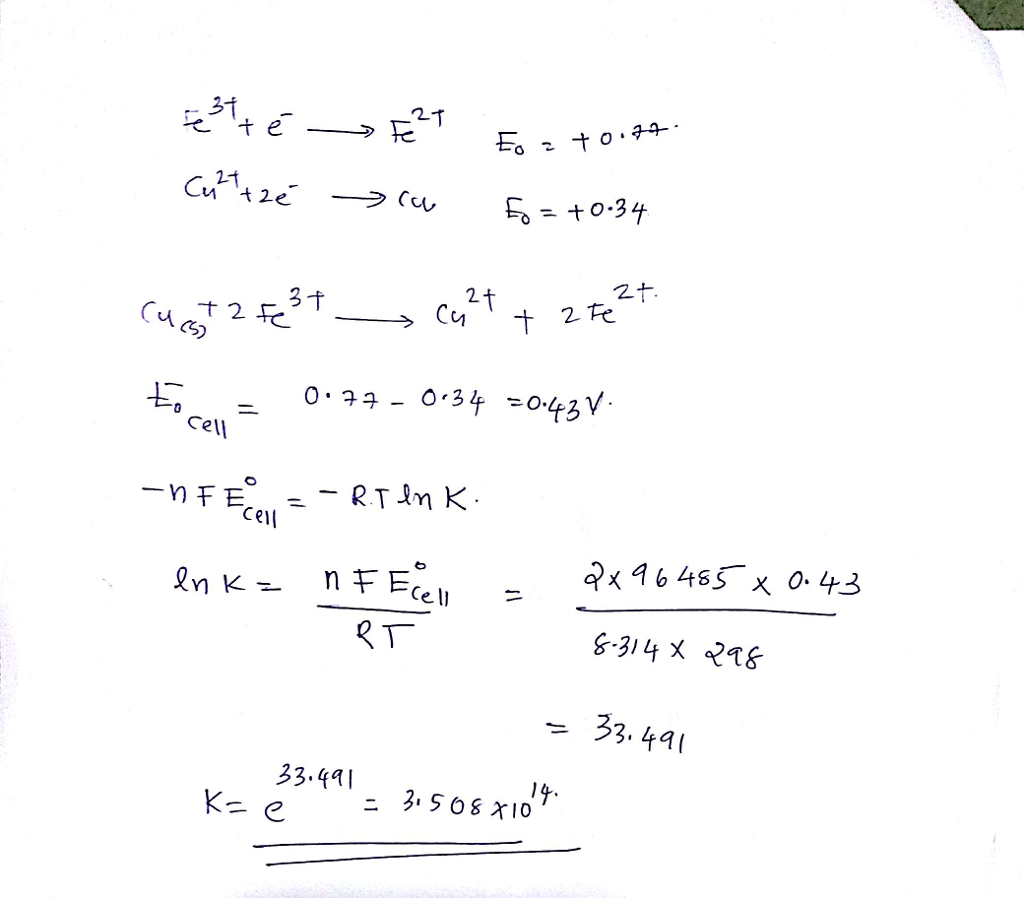# Question & Answer: Calculate the equilibrium constant K for the following reaction at 25 degree C from standard…..Calculate the equilibrium constant K for the following reaction at 25 degree C from standard electrode potentials Fe_(aq)^3+ + Cu_(s) rightarrow Fe_(aq)^2+ + Cu_(aq)^2+ (unbalanced)